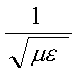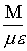Electrodynamic Structure of Space - Part 1 The complexity of nothing, by Steven J. Smith

1.1
Introduction:
Space plays a central role in all electrodynamic interactions.  Both electric and magnetic fields are propagated through the medium of space.  Today, everyone understands that space has a fixed propagation velocity, the speed of light "C".  Less understood or appreciated is the causes of, and implications derived from, this fixed and finite velocity.  Purists will strenuously object to my use of "fixed" to describe the velocity of light, the term they prefer is "isotropic".  However, as even the purists will concede, from a strictly localized point of view, the speed of light does appear as fixed.

1.2.1
Equivalent Circuits:
In electrical engineering we are taught that any unknown electro-magnetic system can be modeled by an equivalent circuit.  The question becomes: "what sort of circuit propagates electro-magnetic waves coherently, and at a fixed velocity?".  The circuit is called a transmission line and a coaxial cable is a good example.  A transmission line consists of a geometric structure that has both inductance [L] and capacitance [C] spread evenly along the length of the line.  This equivalent circuit can be further simplified by considering both inductance per length and capacitance per length to be lumped sum.

1.2.2
Propagation Velocity:
A simple equation exists to determine the propagation velocity of any transmission line.
 [Eq.1]    V =Where:
 V = propagation velocity in meters per second.= inductance in Henries per meter.= capacitance in Farads per meter.

1.2.3
The Speed of Light:
If we build an inductor with empty space for the core, or a capacitor with empty space for the dielectric, the measured values of inductance or capacitance are NOT zero.  When we plug the measured values of inductance and capacitance (per meter) into Eq.1, the resultant propagation velocity is that of light in empty space, "C".  Therefore, empty space behaves just like our physical transmission line equivalent circuit in terms of propagation velocity.

1.2.4
Series or Parallel:
Inductors and capacitors (LC networks) may be connected in either series or parallel to form a transmission line.  When connected in parallel, the DC electrical resistance is near zero.  When connected in series, the DC electrical resistance is close to infinite.  The DC electrical resistance of free space is infinite (below the break down potential).  Therefore space is a series connected LC network.

1.2.5
Thermal Dissipation:
Free space differs from a physical transmission line in one important detail.  Free space has no internal electrical resistance.  It behaves as if constructed of super conducting materials and loss less dielectric and paramagnetic materials.  An electro-magnetic signal in space may be attenuated by the inverse square rule, but never dissipates into random thermal energy.  Therefore space is capable of transmitting electro-magnetic signals or waves without any distance limitation.

1.3.1
Dielectric Materials:
Many non-conductive materials will exhibit dielectric properties to a greater or lesser degree.  The basis for this response to applied electrical fields is polarization of the electric dipoles contained in the material.  The electric dipoles are aligned by the applied electric field, and produce a counter field, in opposition to the applied field.  Materials that are easily polarized produce a greater dielectric response.  All capacitors contain some form of dielectric material.

1.3.2
Paramagnetic Materials:
Any material with un-paired electron spins will exhibit magnetic properties to a greater or lesser extent.  These un-paired electrons will spin align (polarize) with the applied magnetic field.  In paramagnetic materials, the polarization disappears when the applied magnetic field is removed.  Materials that are easily polarized produce a greater paramagnetic response.  All inductors contain some form of paramagnetic material.

1.3.3
Structural Requirements of Space:
Space is a (3 dimensional) transmission line (1.2.1 ), composed of series connected LC networks (1.2.4), that are loss less (1.2.5), and has a propagation velocity set by it's electrical inductance and capacitance (1.2.2 & 1.2.3) as determined by it's dielectric and paramagnetic properties (1.3.1 & 1.3.2).  What possible structure could satisfy this set of requirements?

1.3.4
The Dirac Sea:
In the 1920's P.A.M. Dirac discovered the quantum equation that fully describes an electron.  This equation has the peculiarity of having two symmetrical solutions, differing by the sign of the electron energy.  The positive energy solution was obviously describing an electron, but what was the negative energy solution describing?  It described the positron or anti-electron, later observed in cloud chamber experiments.  Dirac later realized that a negative energy solution implied the existence of a vast sea of electron/positron pairs that acted as the substrate for all real (observable) electrons and positrons.  Observable electrons and positrons are nothing more than particles pulled lose from this Dirac sea, and represent "defects" (unpaired particles) in the sea, much the same way as electrons and holes arise from intentional defects in a semiconductor material.  Each observable particle has a mass equal to the energy required to liberate (pull) the particle from the Dirac sea, and each particle will give up this energy of mass, when it is once more "paired" with it's anti-particle and "falls" back into the sea.  These particle pairs constitute dipoles, and I shall use this term interchangeably with particle/anti-particle pairs.

1.3.5
Composition of Space:
The Dirac sea satisfies all structural requirements of space (1.3.3).  Particle/anti-particle pairs behave as dipoles and will exhibit both required dielectric and paramagnetic properties.  The binding energy of these particle pairs is responsible for the non-conductive nature (infinite DC electrical resistance) of space.  Further, the particle pairs are in a negative energy state, and there is no mechanism by which the pairs can dissipate energy, consequently electro-magnetic waves are propagated without loss.  And since observable particles (real electrons, etc.) are nothing more than un-paired defects in the sea, these particles are free to move in an unimpeded fashion throughout the sea (space).

1.4.1
Uncertainty principle
Observable (real) particles may exchange energy with their Dirac sea counterparts.  In this manner, a real electron falls back into the sea, and a nearby sea electron becomes observable (real).  The consequence of such exchanges is an uncertainty in the observable particle's kinetic state.  Therefore no observable particle is ever truly at rest, and all observable particles possess a zero point energy.

1.4.2
Temperature of Empty Space:
In the absence of electro-magnetic fields, the Dirac sea particle pairs must be completely random in their orientation, because any non-random orientation would give rise to an electro-magnetic field.  This random orientation represents a state of maximum entropy (disorder) and therefore an infinite temperature.  The condition is meta-stable since infinite temperature implies infinite energy, and the sea would "boil" particles into (observable) existence thereby creating electro-magnetic fields, and lowering the temperature of the sea.

1.4.3
Density of Space:
Propagation velocity of electro-magnetic fields is higher in space than in any physical transmission line.  Consequently the dielectric and paramagnetic response of space must be smaller than any physical material, since propagation velocity is inversely proportional to the square root of the product term of inductance and capacitance (Eq. 1).  Or stated another way, the particle/anti-particle pairs in the sea are more tightly bound than the equivalent particles in real (observable) matter.  Therefore space has a higher density than matter!

1.4.4
Matter/Energy Equivalence:
We are now in a position to reformulate Einstein's famous equation E = MC2 by substituting equation Eq. 1 (above) for C2.  The result is:

 [Eq. 2]    E =The implications are nothing short of staggering.  Both matter and energy are electrodynamic in nature.  Further, the values for dielectric and paramagnetic polarization of space determine the ratio of equivalence!

1.4.5
Conclusions:
That space is an electro-magnetic transmission line (1.2.1), composed of dipoles or particle/anti-particle pairs (1.3.5).  That propagation velocity is set by the inductive and capacitive values of these particle pairs (1.2.3).  That observable particles (matter) exist as un-paired defects in the Dirac sea (1.3.4).  That quantum uncertainty arises from interactions between observable particles and the Dirac sea particle/anti-particle pairs (1.4.1).  That space has a higher density than matter (1.4.3).  And most surprising of all, both matter and energy arise from an electrodynamic foundation (1.4.4).  In part 2 we shall consider relativistic effects, curved space and expansion of the universe in light of our new understanding.

End
Electrodynamic Structure of Space - Part 1

 Home